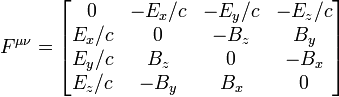### Lorentz transformation of Electron’s field

The only relativistic theory I know for the field of a moving point charge is by applying a Lorentz boost Aμα to the Electromagnetic tensor:.
We choose c=1.

Aμα =
 C S 0 0 S C 0 0 0 0 1 0 0 0 0 1
where C = cosh φ and S = sinh φ .
φ is the ‘rapidity’. The tensor in the boosted frame is F'μν = AμαAνβ Fαβ.

For a motionless point change at the origin sufficient to produce a field of magnitude 1 at distance 1 the field is:
Fαβ = (x2 + y2 + z2)−3/2
 0 −x −y −z x 0 0 0 y 0 0 0 z 0 0 0
Consulting this result:
F'μν = (x2 + y2 + z2)−3/2
 0 −x −Cy −Cz x 0 −Sy −Sz Cy Sy 0 0 Cz Sz 0 0
See this for calculation of F'01. N.B. the coordinates x, y and z are still those of the point charge’s frame!
t = Ct' − Sx', x = Cx' − St', y = y', z = z'.
and we have:
F'μν = ((Cx' − St')2 + y'2 + z'2)−3/2
 0 −(Cx' − St') −Cy' −Cz' Cx' − St' 0 −Sy' −Sz' Cy' Sy' 0 0 Cz' Sz' 0 0
The magnetic field is everywhere perpendicular both to the velocity and the displacement vector from the observer to the charge. The point charge is traveling in the x direction. Note that the x component of the magnetic field (F32) is 0 everywhere. At the event (t', x', y', z') = (0, 0, 0, 1) the electric field is (0, 0, C) and the magnetic field is (0, S, 0). At the event (0, 1, 0, 0) the electric field is (C−2, 0, 0) and the magnetic field is (0, 0, 0).